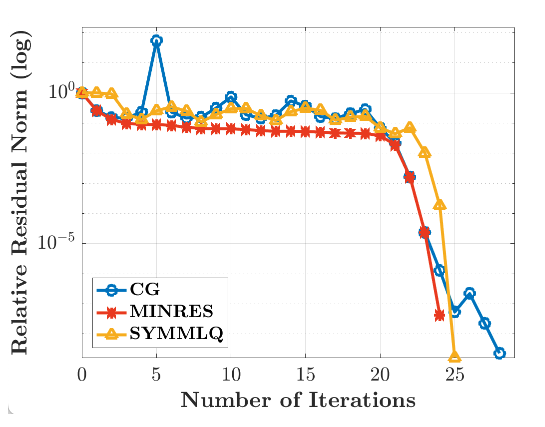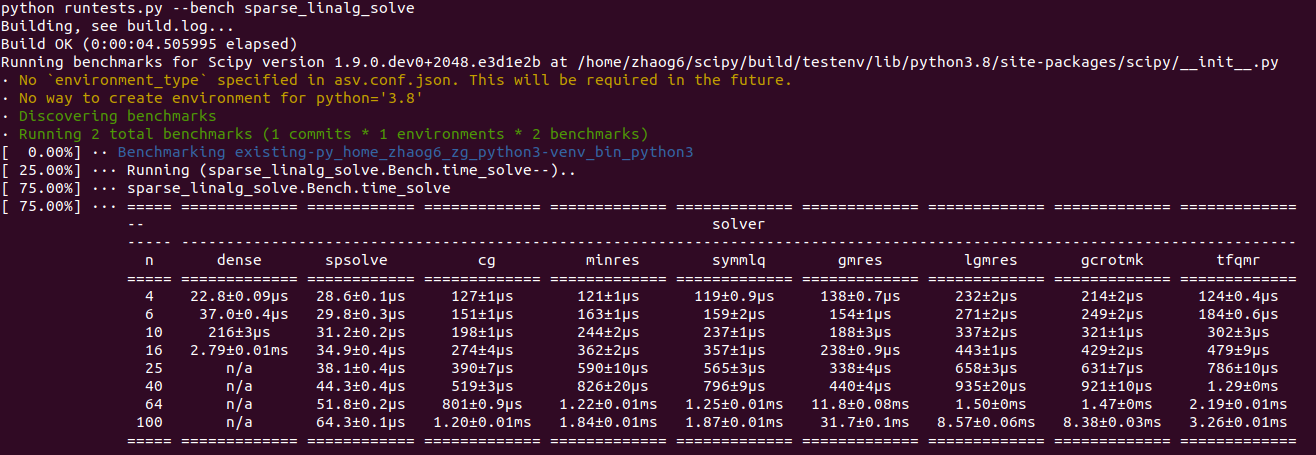Decoding Symmetric Indefinite Matrices In PythonData scientist

## Table of content

• What are symmetric indefinite matrices andwhere are they used?
• Implementing symmetric indefinite matrices (SYMMQL) in python
• Comparing convergence rate of SYMMLQ, CG (Conjugate Gradient), and MINRES by solving the following classical symmetric indefinite system

## What are symmetric indefinite matrices and where are they used?

A matrix is symmetric indefinite if it is symmetric and has both positive and negative eigenvalues. Symmetric Indefinite matrices are a crucial class of matrices since its used for Fine-Tuning in-built algorithms or creating one from scratch. Available in many applications. To name a few: this class of matrices appears in Newton’s methods for the unconstrained/constrained optimization algorithms, penalty function, methods for nonlinear programming, and in discretized incompressible Navier{Stokes equations}. Apart from arising intrinsically in applications, symmetric indefinite matrices are also created from definite ones because of errors in measuring or computing the matrix elements.

As of now, there’s no method of directly dealing with Symmetric Indefinite matrices in Python. People either use the (MINRES) Minimal Residual method or (LSQR) Least Square Root method for working/manipulating symmetric indefinite matrices which is very inconvenient for users. This was a Major deficiency of Python as it cannot meet the demands of professionals who use symmetric indefinite matrices in mechanics, computer science, or ML researchers who find innovative ways to enhance current systems to the next level.

## Implementing symmetric indefinite matrices (SYMMQL) in python

SYMMQL is widely used in programming languages like MATLAB, PETSc, Trilinos, and PyKrylov. For python, The idea of implementing a method(SYMMQL) that keeps up the pace and doesn’t compromise the optimization was initially proposed by C.C.Paige and M.A.Saunders back in 1998. But due to some unknown reasons, It was not carried out. By the way, here There is a Python version of this algorithm, but the library seems to be deprecated, and also it is licensed under LGPL, Hence it was not adopted in python as well.

This year(2022) Gang Zhao picked up this idea and made a PR to make this implementation. But I think the PR is not fully adapted because the following tests were conducted to merge this method into SciPy. However, 13 out of 23 failed : See the tests Comparing convergence rate of SYMMLQ, CG (Conjugate Gradient), and MINRES by solving the following classical symmetric indefinite system

Here’s some prototype code:

``````    import numpy as np
from scipy import sparse

# Building coefficient matrix `A`
n = 50
mu = np.sqrt(3.)
data = np.zeros((3, n))
data[0, :] = 2.
data[1, :] = -1.
data[2, :] = -1.
sp = sparse.spdiags(data,[0, -1, 1],
n, n, format='csr')
A = sp @ sp
muI = mu * sparse.eye(n)
A -= muI

# Building R. H. S. `b`
b = np.ones(n)

``````

The convergence rate is as follows:And CPU time is as follows:For a symmetric indefinite system, compared with CG, SYMMLQ has a smoother convergence curve and better convergence rate. I believe SYMMLQ can replace MINRES to get almost the same performance.

## Conclusion

There’s almost no information available on the internet regarding symmetric indefinite matrices. There are only scholarly articles written decades ago. This topic is very underrated compared to other concepts of ML or computer science. I presume the main reason for that is because it requires substantial knowledge of Linear Algebra + computer science. I hope there will be someone taking the Big leap forward in this.